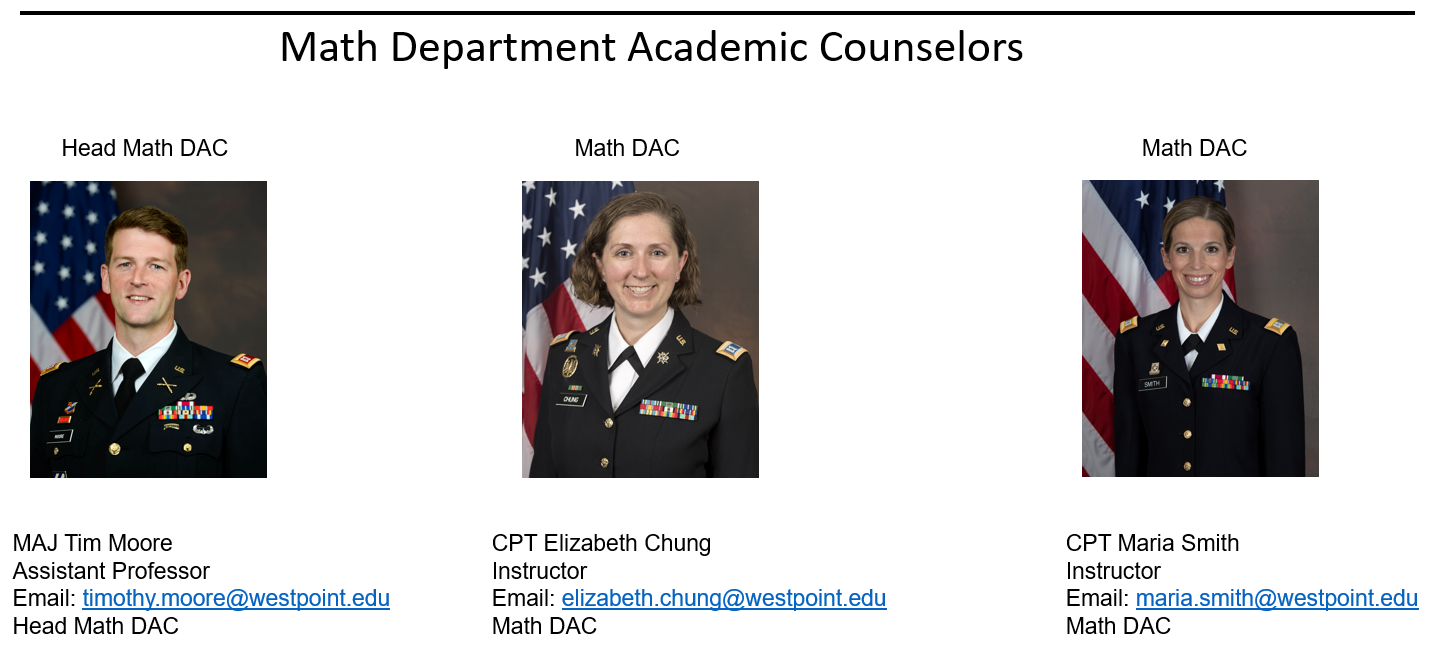# Mathematical Sciences

The mathematical sciences embody those areas of mathematics which have strong interdependence with other disciplines. Their purpose is to clarify concepts and describe scientific phenomena through symbolic language and the rules for its use. Its scope spans the total breadth of knowledge that is capable of being quantified. The full process of the mathematical sciences has expanded from their historical ties with the physical sciences to now include areas such as:  the biological, sociological, behavioral, and computer sciences; operations research; and all engineering fields.

## Military Applications of Mathematics

Mathematics and the ability to use it are particularly important to Army officers, who are leading Soldiers in a complex and increasingly technical world. A successful career depends upon one’s ability to assimilate knowledge from many fields and to apply it to a wide variety of social and technical problems. Mathematics is the common vehicle of thought and expression that enables the leader to model and solve quantifiable problems whether at the platoon or at the Department of the Army level.### Student Outcomes

The student outcomes of the mathematical sciences major include:

1. Demonstrate competence in modeling physical, informational, and social phenomena by:
• Identifying and articulating assumptions, metrics and constraints.
• Applying appropriate solutions techniques.
• Interpreting results within the appropriate context.
2. Argue and inquire soundly and rigorously; become independent questioners and learners.
3. Achieve mathematical proficiency in breadth and depth.
• Understand and apply theorems and algorithms.
• Understand and apply analytical methods.
• Understand and apply numerical methods.
• Understand and apply graphical methods.
• Understand discrete and continuous structures and processes.
4. Communicate mathematics, both orally and in writing.
5. Use technology to model, visualize, and solve complex problems.
6. Develop attitudes – habits of mind:
• Creative and curious.
• Experimental disposition.
• Critical thinking and reasoning.
• Commitment to life-long learning.
7. Understand the role of mathematical sciences (in our world) by analyzing applied problems through disciplinary, multidisciplinary, and interdisciplinary approaches.

### Program of Study

The Mathematical Sciences Major offers abundant opportunities for study in a broad range of mathematical subjects. Courses such as differential equations, algebra, mathematical modeling, analysis, numerical computation, statistics, and linear optimization provide a sound mathematical foundation in the science and engineering fields. In addition, follow-on courses such as algebra, analysis, combinatorics, and advanced individual study provide both depth in understanding the foundations of mathematical theory, as well as opportunity for study and research in a selected subject. Whenever possible, the use of technology is emphasized to extend the knowledge required for the consideration of realistic and challenging problems of today's world.

Cadets interested in studying mathematics or the application of mathematics can pursue a degree in Mathematical Sciences, Mathematical Sciences Studies, or Mathematical Sciences with Honors. All cadets must complete 22 core courses and complete any one of the seven 3-course engineering sequences.

The Mathematical Sciences Major requires an additional 16 courses (10 required and 6 elective) for a total of 41 academic courses.

Mathematical Sciences Studies Major requires an additional 11 courses in the major (8 required and 3 electives) for a total of 40 academic courses. MA387 and MA491 are not required but can be taken as electives.

### Honors Program

The Mathematical Sciences offers an honors program. In order to receive a Mathematical Sciences Major with Honors a cadet is must:

• Complete two courses MA498 Senior Thesis I and MA499 Senior Thesis II in place of MA491
• Graduate with an APSC > 3.0 in core academic program courses
• Graduate with an APSC > 3.5 in mathematical sciences major courses

### Required Courses

Course No. Course Title

#### Mathematical Core (7 of 7)

MA363 Vector Calculus and Ordinary Differential Equations
MA371 Linear Algebra
MA383 Foundations of Mathematics
MA386 Introduction to Numerical Analysis
MA387 Mathematical Analysis I
MA391 Mathematical Modeling
MA491 Research Seminar in Applied Mathematics
##### Statistics Elective (1 of 2)
MA376 Applied Statistics
MA476 Mathematical Statistics
##### Integrative Experience (1 of 2)
MA490 Applied Problems from Math, Science, and Engineering
XX490 Another Department’s Integrative Experience
##### Complementary Support Course (Required)
IT305 Military IT Systems

### Elective Courses

Course No. Course Title

#### Math Electives (Choose 2)

MA372 Introduction to Discrete Mathematics
MA376 Applied Statistics
MA381 Nonlinear Optimization
MA385 Chaos and Fractals
​MA388 Sabermetrics
​​MA394 ​Fundamentals of Network Science
MA396 Numerical Methods for the Solution of Differential Equations
MA461 Graph Theory and Networks
MA462 Combinatorics
MA464 Applied Algebra with Cryptology
MA466 Abstract Algebra
MA476 Mathematical Statistics
MA481 Linear Optimization
MA484 Partial Differential Equations
MA485 Applied Complex Analysis
MA487 Mathematical Analysis II
MA488 Special Topics in Mathematics
MA489 Advanced Individual Study In Mathematics
MA493A Operational Calculus and Transforms
MA493B Real Variable Theory
MA493C Topics in Numerical Analysis
MA493D Introduction to Topology
MA493E Topics in Analysis
##### Science Depth (Choose 1)
CH102 Chemistry II
CH275 Biology
PH206 Physics II
##### Complementary Support Course (CSC) Thread (Choose 2 Courses in any one of these Topics)
8 Course Options Computational Mathematics Tools
5 Course Options Finance Applications
​10 Course Options Cyber Applications
16 Course Options Engineering Applications
​4 Course Options Network Science
​4 Course Options Applied Statistics